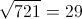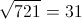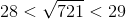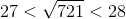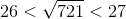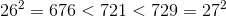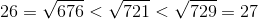ISEE Middle Level Math : How to find the square root

Example Questions

Example Question #101 : How To Find The Square Root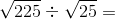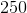Explanation:

First, find the square root: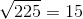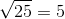Then, solve: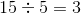Example Question #102 : How To Find The Square Root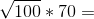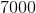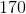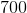Explanation:

First, find the square root: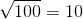Then, solve: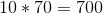Example Question #103 : How To Find The Square Root

Let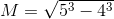.

Then which of the following statements is correct?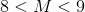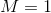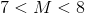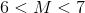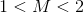Explanation: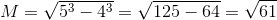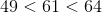Therefore: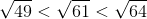Example Question #104 : How To Find The Square Root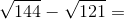Explanation:

First, find the sqaure root: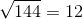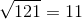Then, solve: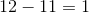Example Question #105 : How To Find The Square Root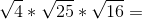Explanation:

First find the square roots: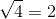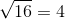Then, solve: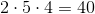Answer:Example Question #106 : How To Find The Square Root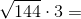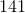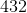Explanation:

First, find the sqaure root:Then, solve: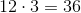Asnwer:Example Question #107 : How To Find The Square Root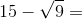Explanation:

First, find the sqaure root: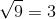Then, solve: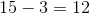Answer:Example Question #108 : How To Find The Square Root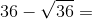Explanation:

First, find the square root: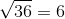Then, solve: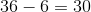Answer:Example Question #109 : How To Find The Square Root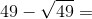Explanation:

First, find the sqaure root: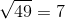Then, solve: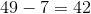Answer:Example Question #110 : How To Find The Square Root

Which of the following is true about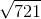?